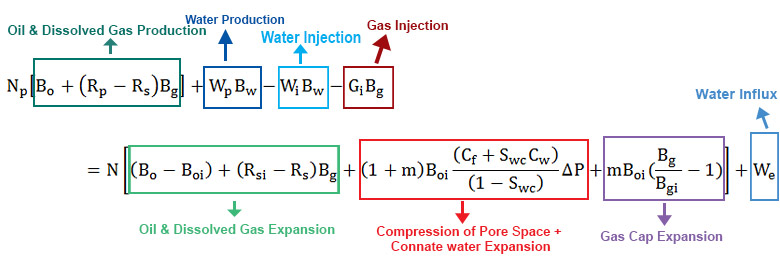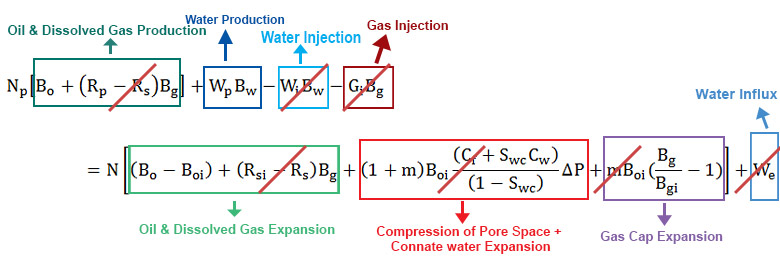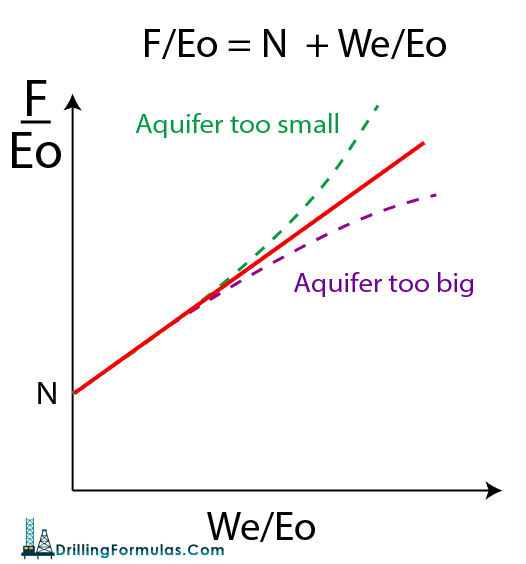# Material Balance for a Water Drive Mechanism

This article will demonstrate a material balance equation in a natural water drive mechanism.  A full material balance equation is shown below:Figure 1 – Full Material Balance Equation

Assumptions

• Reservoir pressure above the bubble point (Pb). Above a bubble point, Rs = Rsi.
• No water injection
• No gas injection
• No gas cap
• Neglect formation and connate water compressibility (Cf and Cw have little effect for a gas cap drive mechanism.)Figure 2 – Material Balance Equation with Assumption for a Natural Water Drive Mechanism

The material balance shown in Figure 2 can be simplified like this:

This can be described in a simple equation (Havlena + Odeh (1963)) like this:

# F/Eo = N + We/Eo

Where;

F = Net fluid production

N = Oil in place

Eo = oil expansion term

We = water influx

The plot is constructed between F/Eo and (We/Eo).Figure 3 – Graphic Solution for Natural Water Drive Mechanism

The interception point on Y-Axis is oil in place (N). If the plot is not a straight line, it indicates that the size of the aquifer is either too small or too big (Figure 3).

Nomenclatures

N = oil initially in place (STOIIP) in reservoir (stb)

Np = cumulative oil production (stb)

Boi = oil volume factor at initial reservoir pressure (rb/stb)

Bo = oil volume factor at current reservoir pressure (rb/stb)

Rsi = solution GOR at initial reservoir pressure (scf/stb)

Rs = solution GOR at current reservoir pressure (scf/stb)

Rp = cumulative produced gas oil ratio (scf/stb)

G = gas volume initially in place (GIIP) in reservoir (scf)

m = ratio of initial gas cap volume to initial oil volume (rb/rb)

Bgi = gas volume factor at initial reservoir pressure (rb/scf)

Bg = gas volume factor at current reservoir pressure (rb/scf)

Swc = connate water saturation (fraction or %)

Cw = water compressibility (1/psi)

Cf = formation (rock) compressibility (1/psi)

Wp = cumulative water production (stb)

We= cumulative water influx from aquifer (rb)

Bw = water volume factor at initial reservoir pressure (rb/stb)

Wi = cumulative water injection (stb)

Gi = cumulative gas injection (scf)

Gp = cumulative gas production (scf)

Eg = gas expansion term (rb/stb)

Eo = oil expansion term (rb/stb)

Efw = formation and connate water expansion term (rb/stb)

References

Abhijit Y. Dandekar, 2013. Petroleum Reservoir Rock and Fluid Properties, Second Edition. 2 Edition. CRC Press.

L.P. Dake, 1983. Fundamentals of Reservoir Engineering, Volume 8 (Developments in Petroleum Science). New impression Edition. Elsevier Science.

Tarek Ahmed PhD PE, 2011. Advanced Reservoir Management and Engineering, Second Edition. 2 Edition. Gulf Professional Publishing.

Share the joy
Tagged , , , , , . Bookmark the permalink.